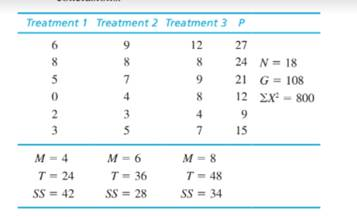Chapter 13, Problem 9PEssentials of Statistics for the B...

8th Edition
Frederick J Gravetter + 1 other
ISBN: 9781133956570

Solutions

Chapter
SectionEssentials of Statistics for the B...

8th Edition
Frederick J Gravetter + 1 other
ISBN: 9781133956570
Textbook Problem

One of the primary advantages of a repealed-measures design, compared to an independent-measures design, is that it reduces the overall variability by removing variance caused by individual differences. The following data are from a research study comparing three treatment conditions. a. Assume that the data are from an independent- measures study using three separate samples, each with n = 6 participants. Ignore the column of P totals and use an independent-measures ANOVA with σ = .05 to test the significance of the mean differences. b. Now assume that the data are from a repealed- measures study using the same sample of n = 6 participants in all three treatment conditions. Use a repealed-measures ANOVA with α = .05 to test the significance of the mean differences. c. Explain why the two analyses lead to different conclusions.a.

To determine

To test: The significance of the mean difference using independent measure ANOVA with α=0.05.

Explanation

Given info:

The data is provided in the question.

 Treatment 1 Treatment 2 Treatment 3 N=18G=108∑X2=800 M=4 M=6 M=8 T=24 T=36 T=48 SS=42 SS=28 SS=34

Calculation:

Step 1:

The null and alternative hypotheses are:

Null hypothesis:

H0:μ1=μ2=μ3,

Where μ1μ2μ3 are the means of the 3 treatments.

Alternate hypothesis:

Ha: At least one treatment mean is different.

Step 2:

Compute the degrees of freedom (df) for the between treatment effects, within treatment effects and total and corresponding sum of squares (SS).

Now, it is known that, for a given sample size, the degrees of freedom (df) is:

df=(number of units)1.

Thus,

dftotal=N1=181=17

The number of treatments, k=3. Thus,

dfbetween=k1=31=2

As within treatments degrees of freedom, dfwithin=dftotaldfbetweentreatment, thus,

dfwithin=dftotaldfbetweentreatment=172=15

With α=0.05 and df=(2,15) critical value from table is CV=3.68

Step 3:

Compute F-ratio.

The total sum of squares is:

SStotal=X2G2N.

The within treatment sum of squares is:

SSwithintreatment=SSinsideeachtreatment.

The within treatment sum of squares is:

SSbetween=T2nG2N

b.

To determine
The significance of mean difference using repeated-measures ANOVA with α=0.05

c.

To determine

To find: Why the results of analysis differ in part a and part b.

Still sussing out bartleby?

Check out a sample textbook solution.

See a sample solution

The Solution to Your Study Problems

Bartleby provides explanations to thousands of textbook problems written by our experts, many with advanced degrees!

Get Started

Convert the expressions in Exercises 8596 radical form. 94(1x)7/3

Finite Mathematics and Applied Calculus (MindTap Course List)

In problems 15-22, simplify by combining like terms. 16.

Mathematical Applications for the Management, Life, and Social Sciences

Domain Find the domain of the function. 69. f(x)=3x4

Precalculus: Mathematics for Calculus (Standalone Book)

Find the constants m and b in the linear function f(x) = mx + b such that f(0) = 2 and f(3) = 1.

Applied Calculus for the Managerial, Life, and Social Sciences: A Brief Approach

Test the series for convergence or divergence. 25. n=1n!en2

Single Variable Calculus: Early Transcendentals

How many distinct five-digit numbers can be made using the digits 1, 2, 2, 2, 7?

Finite Mathematics for the Managerial, Life, and Social Sciences

For f (x) = sinh x, f (x) = a) cosh2 x b) sinh2 x c) tanh x d) sinh x

Study Guide for Stewart's Single Variable Calculus: Early Transcendentals, 8th